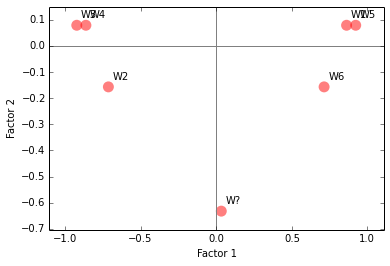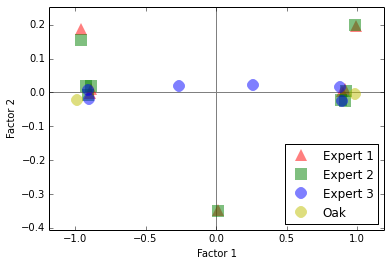mca - Burgundies Example¶

This example demonstrated capabilities of mca package by reproducing results of Multiple Correspondence Analysis, Hedbi & Valentin, 2007.

In :
import mca
import pandas as pd
import numpy as np

np.set_printoptions(formatter={'float': '{: 0.4f}'.format})
pd.set_option('display.precision', 5)
pd.set_option('display.max_columns', 25)

For input format, mca uses DataFrame from pandas package.

Here we use pandas to load CSV file with indicator matrix $X$ of categorical data with 6 observations, 10 variables and 22 levels in total. We also set up supplementary variable $j_{sup}$ and supplementary observation $i_{sup}$.

In :
sep=',', skiprows=1, index_col=0, header=0)
X = data.drop('oak_type', axis=1)
j_sup = data.oak_type
i_sup = np.array([0, 1, 0, 1, 0, .5, .5, 1, 0, 1, 0, 0, 1, 0, .5, .5, 1, 0, .5, .5, 0, 1])
ncols = 10

X.shape, j_sup.shape, i_sup.shape
Out:
((6, 22), (6,), (22,))

Table 1¶

"Data for the barrel-aged red burgundy wines example. “Oak Type" is an illustrative (supplementary) variable, the wine W? is an unknown wine treated as a supplementary observation." (Hedbi & Valentin, 2007)

In :
src_index = (['Expert 1'] * 7 + ['Expert 2'] * 9 + ['Expert 3'] * 6)
var_index = (['fruity'] * 2 + ['woody'] * 3 + ['coffee'] * 2 + ['fruity'] * 2
+ ['roasted'] * 2 + ['vanillin'] * 3 + ['woody'] * 2 + ['fruity'] * 2
+ ['butter'] * 2 + ['woody'] * 2)
yn = ['y','n']; rg = ['1', '2', '3']; val_index = yn + rg + yn*3 + rg + yn*4
col_index = pd.MultiIndex.from_arrays([src_index, var_index, val_index],
names=['source', 'variable', 'value'])

table1 = pd.DataFrame(data=X.values, index=X.index, columns=col_index)
table1.loc['W?'] = i_sup
table1['','Oak Type',''] = j_sup

table1
Out:
source Expert 1 Expert 2 Expert 3
variable fruity woody coffee fruity roasted vanillin woody fruity butter woody Oak Type
value y n 1 2 3 y n y n y n 1 2 3 y n y n y n y n
W1 1 0 0 0 1 0.0 1.0 1 0 0 1 0 0 1 0.0 1.0 0 1 0.0 1.0 0 1 1
W2 0 1 0 1 0 1.0 0.0 0 1 1 0 0 1 0 1.0 0.0 0 1 1.0 0.0 1 0 2
W3 0 1 1 0 0 1.0 0.0 0 1 1 0 1 0 0 1.0 0.0 0 1 1.0 0.0 1 0 2
W4 0 1 1 0 0 1.0 0.0 0 1 1 0 1 0 0 1.0 0.0 1 0 1.0 0.0 1 0 2
W5 1 0 0 0 1 0.0 1.0 1 0 0 1 0 0 1 0.0 1.0 1 0 0.0 1.0 0 1 1
W6 1 0 0 1 0 0.0 1.0 1 0 0 1 0 1 0 0.0 1.0 1 0 0.0 1.0 0 1 1
W? 0 1 0 1 0 0.5 0.5 1 0 1 0 0 1 0 0.5 0.5 1 0 0.5 0.5 0 1 NaN

MCA¶

Let's create two MCA instances - one with Benzécri correction enabled (default) and one without it. Parameter ncols denotes number of categorical variables.

In :
mca_ben = mca.MCA(X, ncols=ncols)
mca_ind = mca.MCA(X, ncols=ncols, benzecri=False)

print(mca.MCA.__doc__)
Run MCA on selected columns of a pd DataFrame.
If the column are specified, assume that they hold
categorical variables that need to be replaced with
dummy indicators, otherwise process the DataFrame as is.

'cols': The columns of the DataFrame to process.
'ncols': The number of columns before dummy coding. To be passed if cols isn't.
'benzecri': Perform Benzécri correction (default: True)
'TOL': value below which to round eigenvalues to zero (default: 1e-4)

Table 2 (L, expl_var)¶

"Eigenvalues, corrected eigenvalues, proportion of explained inertia and corrected proportion of explained inertia. The eigenvalues of the Burt matrix are equal to the squared eigenvalues of the indicator matrix; The corrected eigenvalues for Benzécri and Greenacre are the same, but the proportion of explained variance differ. Eigenvalues are denoted by λ, proportions of explained inertia by τ (note that the average inertia used to compute Greenacre’s correction is equal to I = .7358)." (Hedbi & Valentin, 2007)

Field L contains the eigenvalues, or the principal inertias, of the factors. Method expl_var returns proportion of explained inertia for each factor, whereas Greenacre corrections may be enabled with parameter greenacre and N limits number of retained factors.

Note that Burt matrix values are not included in the following table, as it is not currently implemented in mca package.

In :
data = {'Iλ': pd.Series(mca_ind.L),
'τI': mca_ind.expl_var(greenacre=False, N=4),
'Zλ': pd.Series(mca_ben.L),
'τZ': mca_ben.expl_var(greenacre=False, N=4),
'cλ': pd.Series(mca_ben.L),
'τc': mca_ind.expl_var(greenacre=True, N=4)}

# 'Indicator Matrix', 'Benzecri Correction', 'Greenacre Correction'
columns = ['Iλ', 'τI', 'Zλ', 'τZ', 'cλ', 'τc']
table2 = pd.DataFrame(data=data, columns=columns).fillna(0)
table2.index += 1
table2.loc['Σ'] = table2.sum()
table2.index.name = 'Factor'

table2
Out:
τI τZ τc
Factor
1 0.8532 0.7110 0.7004 0.9823 0.7004 0.9519
2 0.2000 0.1667 0.0123 0.0173 0.0123 0.0168
3 0.1151 0.0959 0.0003 0.0004 0.0003 0.0004
4 0.0317 0.0264 0.0000 0.0000 0.0000 0.0000
Σ 1.2000 1.0000 0.7130 1.0000 0.7130 0.9691

The inertia is simply the sum of the principle inertias:

In :
mca_ind.inertia, mca_ind.L.sum(), mca_ben.inertia, mca_ben.L.sum()
Out:
(1.1999999999999997,
1.1999999999999997,
0.71302972718038071,
0.71302972718038071)

Table 3 (fs_r, cos_r, cont_r, fs_r_sup)¶

"Factor scores, squared cosines, and contributions for the observations (I-set). The eigenvalues and proportions of explained inertia are corrected using Benzécri/Greenacre formula. Contributions corresponding to negative scores are in italic. The mystery wine (Wine ?) is a supplementary observation. Only the first two factors are reported." (Hedbi & Valentin, 2007)

Firstly, we once again tabulate eigenvalues and their proportions. This time only for the first two factors and as percentage.

In :
data = np.array([mca_ben.L[:2],
mca_ben.expl_var(greenacre=True, N=2) * 100]).T
df = pd.DataFrame(data=data, columns=['cλ','%c'], index=range(1,3))
df
Out:
%c
1 0.7004 95.1889
2 0.0123 1.6779

Factor scores, squared cosines, and contributions for the observations are computed by fs_r, cos_r and cont_r methods respectively, where r denotes rows (i.e. observations). Again, N limits the number of retained factors.

Factor scores of supplementary observation $i_{sup}$ is computed by method fs_r_sup.

Note that squared cosines do not agree with those in the reference. See issue #1.

In :
fs, cos, cont = 'Factor score','Squared cosines', 'Contributions x 1000'
table3 = pd.DataFrame(columns=X.index, index=pd.MultiIndex
.from_product([[fs, cos, cont], range(1, 3)]))

table3.loc[fs,    :] = mca_ben.fs_r(N=2).T
table3.loc[cos,   :] = mca_ben.cos_r(N=2).T
table3.loc[cont,  :] = mca_ben.cont_r(N=2).T * 1000
table3.loc[fs, 'W?'] = mca_ben.fs_r_sup(pd.DataFrame([i_sup]), N=2)

np.round(table3.astype(float), 2)
Out:
Wine W1 W2 W3 W4 W5 W6 W?
Factor score 1 0.86 -0.71 -0.92 -0.86 0.92 0.71 0.03
2 0.08 -0.16 0.08 0.08 0.08 -0.16 -0.63
Squared cosines 1 0.99 0.95 0.99 0.99 0.99 0.95 NaN
2 0.01 0.05 0.01 0.01 0.01 0.05 NaN
Contributions x 1000 1 176.68 120.99 202.33 176.68 202.33 120.99 NaN
2 83.33 333.33 83.33 83.33 83.33 333.33 NaN

Table 4 (fs_c, cos_c, cont_c, fs_c_sup)¶

"Factor scores, squared cosines, and contributions for the variables (J-set). The eigenvalues and percentages of inertia have been corrected using Benzécri/Greenacre formula. Contributions corresponding to negative scores are in italic. Oak 1 and 2 are supplementary variables." (Hedbi & Valentin, 2007)

Computations for columns (i.e. variables) are analogous to those of rows. Before the supplementary variable factor scores can be computed, $j_{sup}$ must be converted from categorical variable into dummy indicator matrix by method mca.dummy.

In :
table4 = pd.DataFrame(columns=col_index, index=pd.MultiIndex
.from_product([[fs, cos, cont], range(1, 3)]))
table4.loc[fs,  :] = mca_ben.fs_c(N=2).T
table4.loc[cos, :] = mca_ben.cos_c(N=2).T
table4.loc[cont,:] = mca_ben.cont_c(N=2).T * 1000

fs_c_sup = mca_ben.fs_c_sup(mca.dummy(pd.DataFrame(j_sup)), N=2)
table4.loc[fs, ('Oak', '', 1)] = fs_c_sup
table4.loc[fs, ('Oak', '', 2)] = fs_c_sup

np.round(table4.astype(float), 2)
Out:
source Expert 1 Expert 2 Expert 3 Oak
variable fruity woody coffee fruity roasted vanillin woody fruity butter woody
value y n 1 2 3 y n y n y n 1 2 3 y n y n y n y n 1 2
Factor score 1 0.90 -0.90 -0.97 0.00 0.97 -0.90 0.90 0.90 -0.90 -0.90 0.90 -0.97 0.00 0.97 -0.90 0.90 0.28 -0.28 -0.90 0.90 -0.90 0.90 0.99 -0.99
2 0.00 0.00 0.18 -0.35 0.18 -0.00 0.00 0.00 -0.00 -0.00 0.00 0.18 -0.35 0.18 -0.00 0.00 -0.00 0.00 -0.00 0.00 -0.00 0.00 0.00 -0.00
Squared cosines 1 1.00 1.00 0.97 0.00 0.97 1.00 1.00 1.00 1.00 1.00 1.00 0.97 0.00 0.97 1.00 1.00 0.97 0.97 1.00 1.00 1.00 1.00 NaN NaN
2 0.00 0.00 0.03 1.00 0.03 0.00 0.00 0.00 0.00 0.00 0.00 0.03 1.00 0.03 0.00 0.00 0.00 0.00 0.00 0.00 0.00 0.00 NaN NaN
Contributions x 1000 1 57.96 57.96 44.37 0.00 44.37 57.96 57.96 57.96 57.96 57.96 57.96 44.37 0.00 44.37 57.96 57.96 5.56 5.56 57.96 57.96 57.96 57.96 NaN NaN
2 0.00 0.00 83.33 333.33 83.33 0.00 0.00 0.00 0.00 0.00 0.00 83.33 333.33 83.33 0.00 0.00 0.00 0.00 0.00 0.00 0.00 0.00 NaN NaN

Figure 1¶

"Multiple Correspondence Analysis. Projections on the first 2 dimensions. The eigenvalues (λ) and proportion of explained inertia (τ) have been corrected with Benzécri/Greenacre formula. (a) The I set: rows (i.e., wines), wine ? is a supplementary element. (b) The J set: columns (i.e., adjectives). Oak 1 and Oak 2 are supplementary elements. (the projection points have been slightly moved to increase readability). (Projections from Tables 3 and 4)." (Hedbi & Valentin, 2007)

Following plots do not introduce anything new in terms of mca package, it just reuses factor scores from Tables 3 and 4. But everybody loves colourful graphs, so...

In :
%matplotlib inline
import matplotlib.pyplot as plt

points = table3.loc[fs].values
labels = table3.columns.values

plt.figure()
plt.margins(0.1)
plt.axhline(0, color='gray')
plt.axvline(0, color='gray')
plt.xlabel('Factor 1')
plt.ylabel('Factor 2')
plt.scatter(*points, s=120, marker='o', c='r', alpha=.5, linewidths=0)
for label, x, y in zip(labels, *points):
plt.annotate(label, xy=(x, y), xytext=(x + .03, y + .03))
plt.show()In :
noise = 0.05 * (np.random.rand(*table4.T[fs].shape) - 0.5)

fig, ax = plt.subplots()
plt.margins(0.1)
plt.axhline(0, color='gray')
plt.axvline(0, color='gray')
plt.xlabel('Factor 1')
plt.ylabel('Factor 2')
ax.margins(0.1)
markers = '^', 's', 'o', 'o'
colors = 'r', 'g', 'b', 'y'
for fscore, marker, color in zip(fs_by_source, markers, colors):
label, points = fscore
ax.plot(*points.T.values, marker=marker, color=color, label=label, linestyle='', alpha=.5, mew=0, ms=12)
ax.legend(numpoints=1, loc=4)
plt.show()